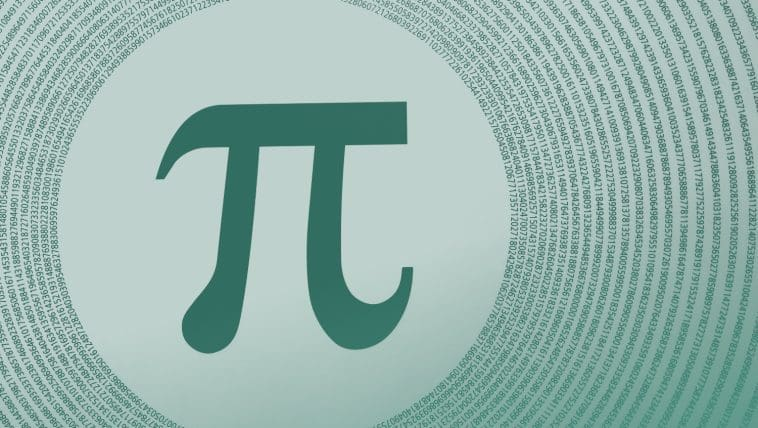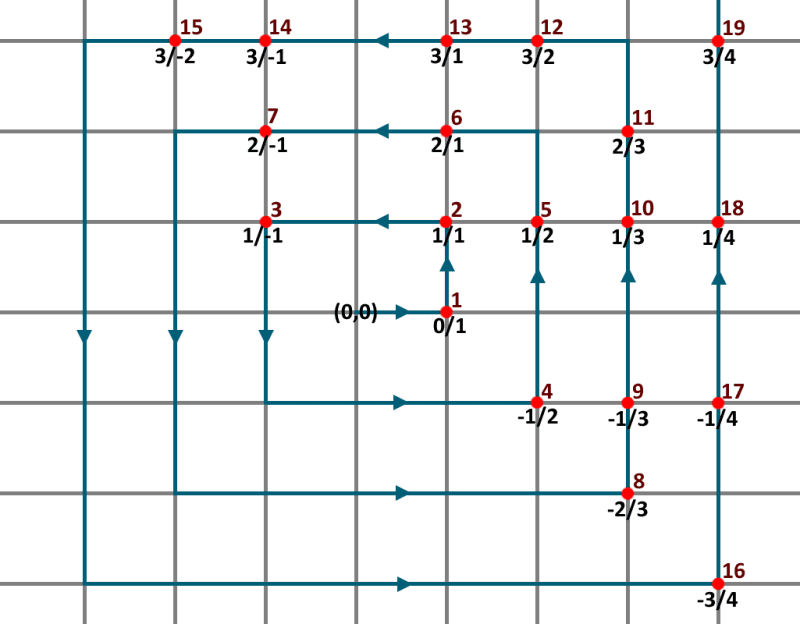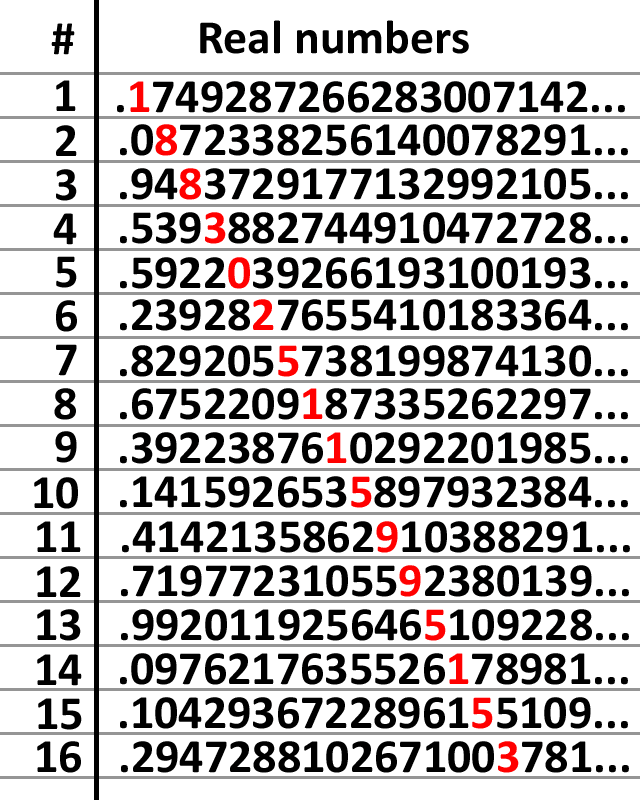# Wrapping our Minds around…Infinity? Part 2[Originally published part of Infinity]

But infinity often yields surprising results.

Amazingly, the number of rational numbers is the same infinity as the counting numbers. A rational number is any number that can be expressed as the ratio of two integers, as in a/b.

It is perhaps surprising and counterintuitive that rational numbers would be the same infinity as the counting numbers. After all, the rational numbers completely contain all the counting numbers plus an infinite quantity of numbers that are in between them.

In fact, there are an infinite number of rational numbers between any two counting numbers. Yet, we can demonstrate from logic that the two sets are the same size. We again do this by showing that there is exactly one positive integer for each rational number.

This can be demonstrated geometrically as follows.In the figure above, starting at the origin, we move to the coordinates (1,0). Let the x-coordinate (1) represent the denominator of a rational number, and the y-coordinate (0) represent the numerator.  So this point represents the rational number 0/1, which is zero. This is the first rational number we have explored, so we label it with counting number 1.

Next, we move up to (1,1), representing the rational number 1/1, which is 1. This is the second rational number we have encountered, so we label it 2.

We then move left two spaces and assign the next counting number, then down two, then right three, and so on, forming a rectangular spiral pattern (as shown in blue), stopping at each pair of integer coordinates.

We skip those coordinates where the denominator would be zero, and we also do not count duplicates. (That is, -2/2 is the same rational number as -1/1, so we do not count it twice.) As we spiral outward, we will eventually hit every possible rational number, since each pair of integer x and y coordinates is eventually crossed.

Furthermore, each rational number will be assigned a positive integer. Thus, there is exactly one positive integer for each rational number. Therefore, the set of rational numbers is the same size as the set of counting numbers.

They are the same infinity!

## Hilbert’s Grand Hotel

The strangeness of infinity is illustrated in a delightful paradox first proposed by David Hilbert in 1924.

Imagine a hotel with an infinite number of rooms — one room for each counting number. An infinite number of guests arrive, and each is assigned to one room in the hotel. The hotel is now fully occupied.

An additional guest shows up and requests a room. We might at first think that no rooms would be available in a fully occupied hotel. And this would indeed be the case for a hotel with a finite number of rooms. However, the hotel manager is able to accommodate the request by asking each guest to move to the next room. That is, the person in Room 1 moves to Room 2, the person in Room 2 moves to Room 3, and so on.

Since there is no “last” room in an infinite hotel, each guest is able to do this, which leaves Room 1 empty and available for the new guest.

But suppose an infinite number of additional people show up the same evening. Each room in the hotel is already occupied, and yet the hotel manager is again able to accommodate the new visitors in the following way. He asks each person already in a hotel room to move to the room that is twice the number of the current room. So the person in Room 1 moves to Room 2, the person in Room 2 moves to Room 4, and so on. This leaves all the odd-numbered rooms unoccupied. And since there are an infinite number of odd rooms, each of the new visitors is assigned to one of these rooms and the hotel is again fully occupied.

## Countable and Uncountable Infinities

The infinity we have discussed so far is countable. Countable means that if you started counting 1,2,3,4, and continued forever, you would eventually hit every positive integer. This is the smallest possible infinity.¹  Its “size” or cardinality is represented by the Hebrew letter aleph with a subscript zero 0), and pronounced “aleph naught.”

However, not all infinities are countable. There are some infinite sets where even if you spent all eternity counting their elements, there would still be an infinite number that you could never count. Uncountable infinities are therefore larger than countable infinities; that is, they have a larger cardinality.

One example of an uncountable infinity is the quantity of real numbers.

The real numbers include both the rational and irrational numbers. In mathematics, an irrational number is one that cannot be expressed as the ratio of two finite integers such as a/b. The decimal expression of an irrational number goes on forever without settling on any infinitely repeating sequence.

Perhaps the most well-known irrational number is pi: the ratio of the circumference to diameter in a circle.  The decimal expression of pi continues forever without a recognizable pattern:

### 3.1415926535897932384626433…

Other irrational numbers include Euler’s number (e) as well as the square root of any integer that is not itself an integer, e.g. the square root of 2.

How can we prove that the set of real numbers is a larger infinity than the countable numbers?

We do this by a reductio ad absurdum. This is the method of demonstrating that a claim is false by assuming (for the sake of argument) that it is true and showing how this would lead to a contradiction. So, we assume for the sake of hypothesis that we can list the real numbers in such a way that each one is assigned to exactly one counting number. To make this more tractable, we will consider only the real numbers between zero and one. If this is a greater infinity than the countable numbers, then so must be the infinity of all the real numbers which include this list.

The order of the real numbers would have to be random because there is an infinite quantity of numbers in between any two real numbers. But let’s assume that each and every real number between 0 and 1 is on this list in the right-hand column of the figure below. And each corresponds to exactly one positive integer in the left-hand column.Now, we are going to construct a real number by the following method.

• Take the first digit of the first number on the right-hand column (shown in red) and pick any number except that one. The first digit of the first number is 1, so let’s pick 2.
• Now look at the second digit of the second number on the list (also in red) and pick any number except that one. So let’s pick 9.
• Then we examine the third digit of the third number and pick a different number, and so on.

If we were to do this forever, we would end up with a real number between zero and one, such as 0.29941362260062624….

However, the number we have constructed cannot be in the right-hand column because it differs by at least one digit in one location from every number on this list! Yet, we stipulated at the outset that the right-hand column contained every real number between zero and one, each corresponding to one positive integer.

We have reached a contradiction since we have constructed a real number between 0 a 1 that is not on this list. Therefore, we now see that such a list cannot exist, because we can always construct a real number that is not on this list by changing one number in each digit from numbers that are on the list.

Therefore, our initial claim (that there can be a list of real numbers with each one corresponding to a positive integer) is disproved. There will always be real numbers that are not on such a list.

Thus, the quantity of real numbers (between zero and one) is greater than the quantity of positive integers. And since the set of all real numbers includes the set of real numbers between zero and one, it too is a larger infinity than the set of positive integers. The set of all real numbers is therefore a non-countable infinity. It is larger than א0In fact, the cardinality of real numbers has been proved to be 2 to the power of א0.

Could the real numbers represent the second smallest infinity? Many mathematicians suspect this is the case, but it is unproven. The concept is called the continuum hypothesis. If it is correct, then the quantity of real numbers is indeed the second smallest infinity and designated as א1. If so, then there is no set with a cardinality between that of the integers and the real numbers.

## Infinite Conclusions

It is truly remarkable that we can reason and draw correct conclusions about infinity.  After all, our minds are finite. Yet, the laws of logic and mathematics by which we reason are useful even in areas that we cannot fully grasp. This is because such laws reflect the thinking of the Lord. And by His grace, He has allowed us to use these laws to reason correctly about things that are infinitely beyond us.

Only the biblical worldview can make sense of this truth. Glory be to God!

## Written by Dr. Jason Lisle

Dr. Jason Lisle is a Christian astrophysicist who researches issues pertaining to science and the Christian Faith. You can find his ministry at Biblical Science Institute.com.
Dr. Lisle double-majored in physics and astronomy with a minor in mathematics at Ohio Wesleyan University. He then went on to obtain a Master’s degree and Ph.D. in astrophysics at the University of Colorado in Boulder. There, he used the SOHO spacecraft to analyze the surface of the sun, and made a number of interesting discoveries, including the detection of giant cell boundaries.
Since then, Lisle has worked in full-time apologetics ministry. He wrote a number of planetarium shows for the Creation Museum, including the popular “Created Cosmos.” Dr. Lisle has authored a number of best-selling books on the topic of creation, including: Taking Back Astronomy, Stargazer’s Guide to the Night Sky, the Ultimate Proof of Creation, Discerning Truth, and Understanding Genesis.